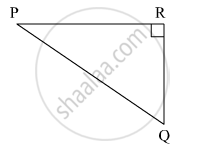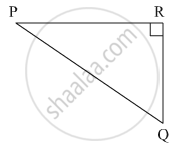Advertisement Remove all ads

# In the Given Fig, ∠ R is the Right Angle of δ Pqr. Write the Following Ratios. (I) Sin P (Ii) Cos Q (Iii) Tan P (Iv) Tan Q - Geometry

Sum

In the given Fig, angle R is the right angle of triangle PQR. Write the following ratios.
(i) sin P (ii) cos Q (iii) tan P (iv) tan QAdvertisement Remove all ads

#### Solution(i) Sin P = " Opposite side of ∠P"/" Hypotenuse " = ["QR"]/["PQ"]

(ii) CosQ = "Adjacent side of ∠Q"/" Hypotenuse " = ["QR"]/["PQ"]

(iii) tan P = " Opposite side of ∠P"/"Adjacent side of ∠P" = ["QR"]/["PR"]

(iv) tan Q = " Opposite side of ∠P"/"Adjacent side of ∠P" = ["PR "]/["QR"]

Is there an error in this question or solution?
Advertisement Remove all ads

#### APPEARS IN

Balbharati Mathematics 2 Geometry 9th Standard Maharashtra State Board
Chapter 8 Trigonometry
Practice Set 8.1 | Q 1 | Page 104
Advertisement Remove all ads
Advertisement Remove all ads
Share
Notifications

View all notifications

Forgot password?